# How to get the cell value based on row and column numbers in Excel?

In the article user will understand the process of generating a cell value by utilizing the row and column numbers in excel. The main purpose of the article is to describe two simple ways to solve the provided task. In the first example, an already defined formula is used, that is by utilizing the INDIRECT value, user can generate the required result. On the other hand, the second example is generating the same cell value by using the VBA code. After writing the VBA code, the user needs to generate a function call from a provided method.

## Example 1: To get the cell value based on row and column numbers in excel by using the user defined formula:

### Step 1:

This worksheet contains three columns. First column with data values for “Name of product”, second column contains data for “Age of product”, and third column contains “sample number”. After that in the H3 cell create header for row with value 3 stored in the I3 cell. similarly, go to cell H4, to create a column header, with value 5 stored in the I4 cell. finally, create H5 cell with header “RESULT”. The resultant value will be stored to the cell I5. Consider below provided snapshot for proper reference: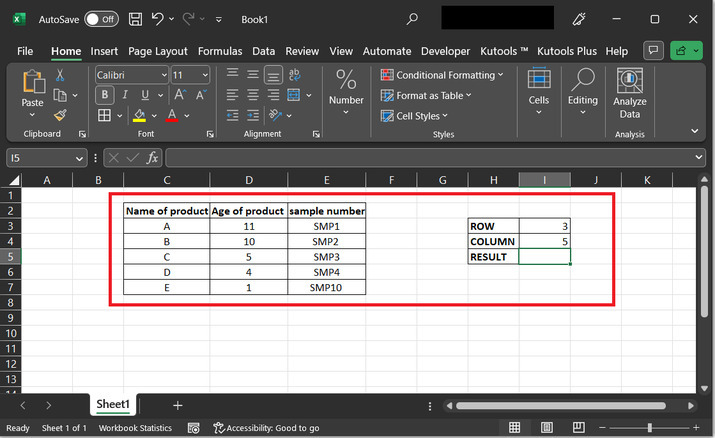### Step 2:

Go to the I5, cell and type or paste the formula “=INDIRECT(ADDRESS(I3,I4))”. Consider proper snapshot provided below for detailed reference −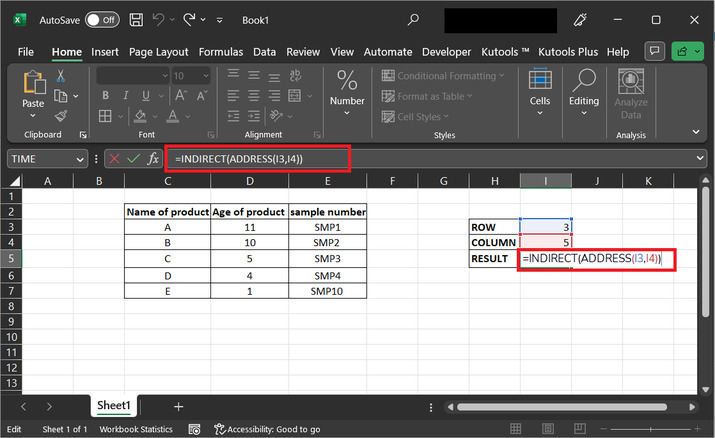### Explanation for above formula:

• The ADDRESS function takes two arguments: the row number and the column number of a cell, and returns a text string that represents the address of that cell in A1-style notation. In this case, the row number is given by the value in cell I3, and the column number is given by the value in cell I4. For example, if I3 contains the value 5 and I4 contains the value 7, then the result of the ADDRESS function would be the text string "G5".

• The INDIRECT function takes a text string argument that represents a reference to a cell or range of cells in Excel, and returns the value of that cell or range. In this case, the text string that represents the cell reference is obtained from the ADDRESS function as described above. So if the result of the ADDRESS function is "G5", then the INDIRECT function will return the value of the cell at column G and row 5.

In summary, the formula INDIRECT(ADDRESS(I3,I4)) returns the value of the cell that is specified by the row and column numbers in cells I3 and I4, respectively. This can be useful in situations where you want to refer to a cell using dynamic row and column numbers that are determined by other cells in the worksheet.

### Step 3:

After typing the formula, press “Enter” key, to obtain the required output result.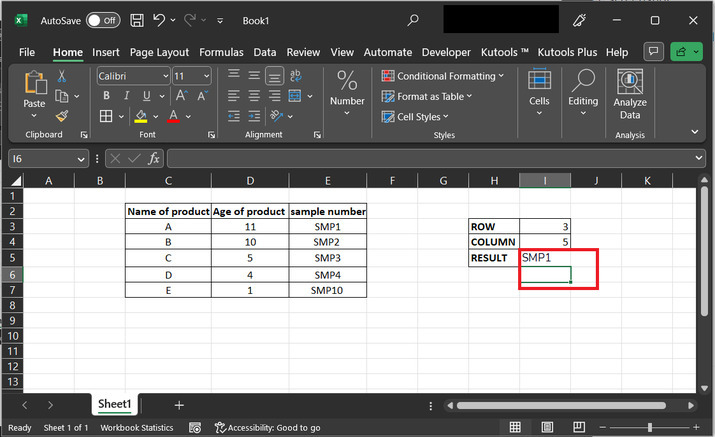The final obtained result contains sample number for 3rd row, and 5th column, that is the generated result is SMP1.

## Example 2: To get the cell value based on row and column numbers in excel by using the VBA code:

### Step 1:

This worksheet contains three columns. First column with data values for “Name of product”, second column contains data for “Age of product”, and third column contains “sample number”. Consider below provided snapshot for proper reference−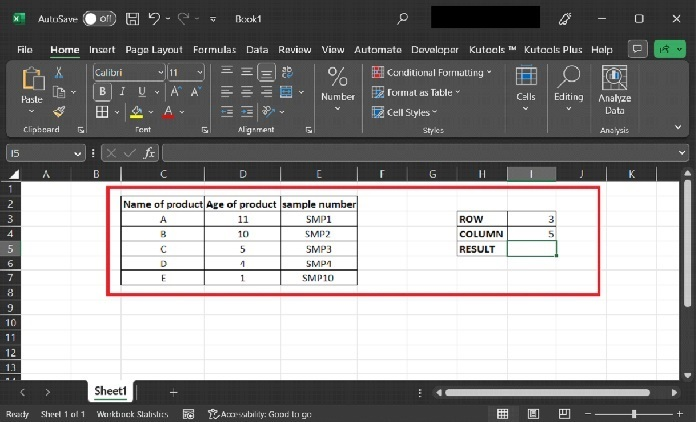### Step 2:

To open the code editor for VBA, go to the “Developer” tab, and choose option for “Visual Basic”, under the code section. Consider below provide snapshot −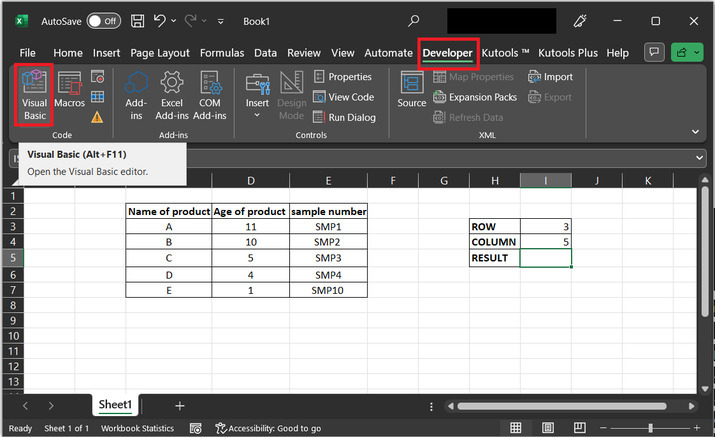### Step 3:

The above step will open a “Microsoft Visual Basic for Applications” dialog box.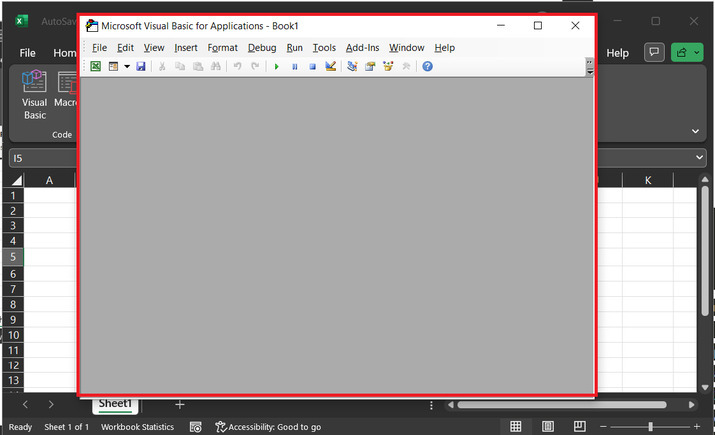### Step 4:

Go to the menu bar and click on “Insert”. After that click on “Module”. Consider below provided snapshot for reference−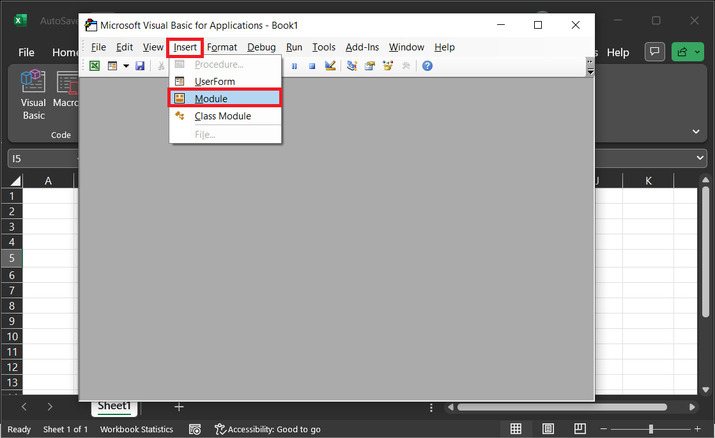### Step 5:

The above step will open a blank code area, as depicted below −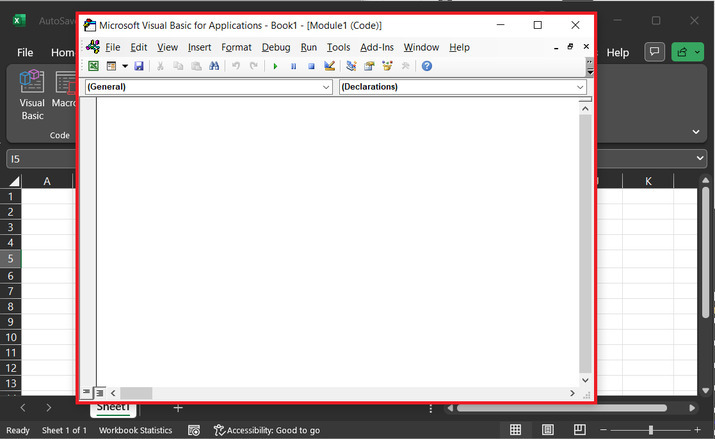### Step 6:

Copy the below-given code to the code window.

' function header
Function get_val(r As Integer, c As Integer)
' retrieving data from a provided location
get_val = ActiveSheet.Cells(r, c)
' end of the function header
End Function


Use proper code indentation to avoid coding errors. The code snapshot is depicted below, use it for complete and precise code writing.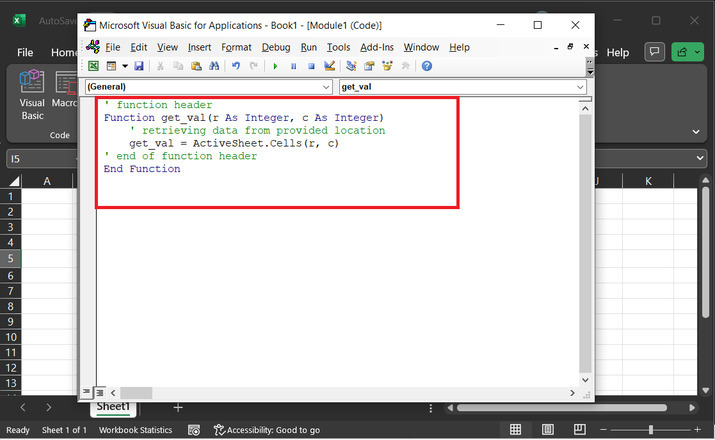### Step 5:

After declaring the function module, press “Alt + Q” key combination to exit the current dialog box. In the excel sheet, write the formula “=get_val(I3,I4)” to generate the possible results. Here, get_val is the name of function, and I3 and I4 are the cell reference for the excel sheet.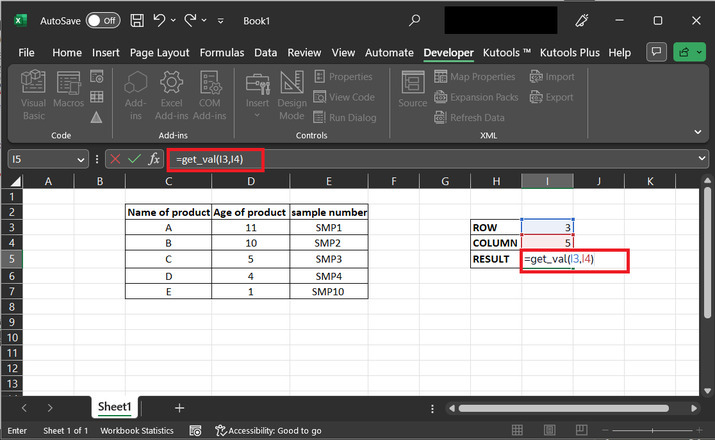### Step 6:

The generated result value is SMP1. Consider below provide image for proper reference −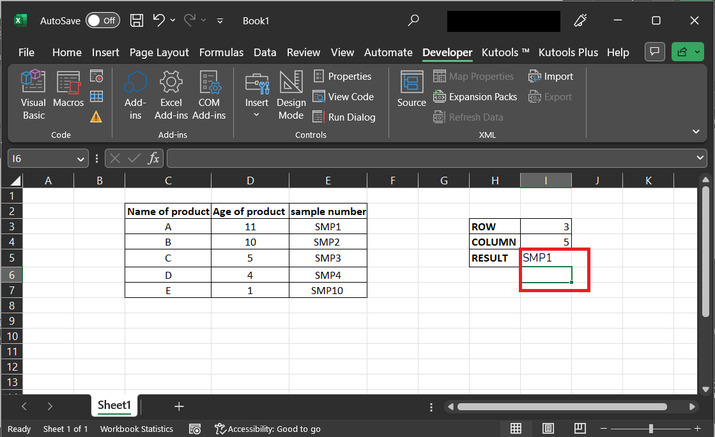## Conclusion

This article contains explanations for two examples. Both examples will perform the same task and can generate the same sample number based on the provided row and column values. The only difference between them is the approach. The first example calculates data by using the formula, while the second example calculates the result based on the provided VBA code, and can work only when the defined function is called with proper parameter values.